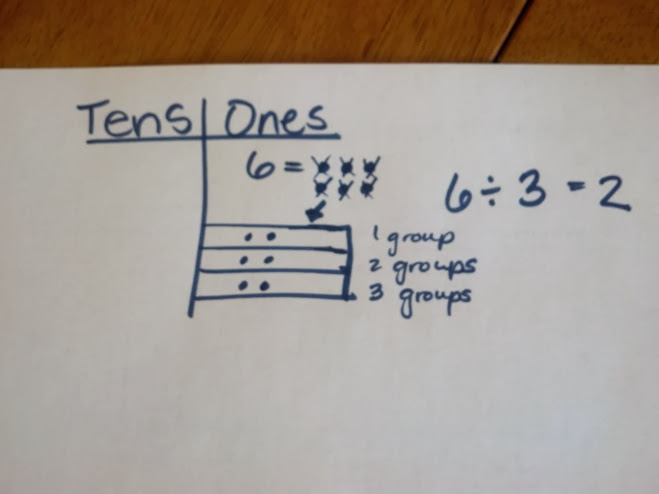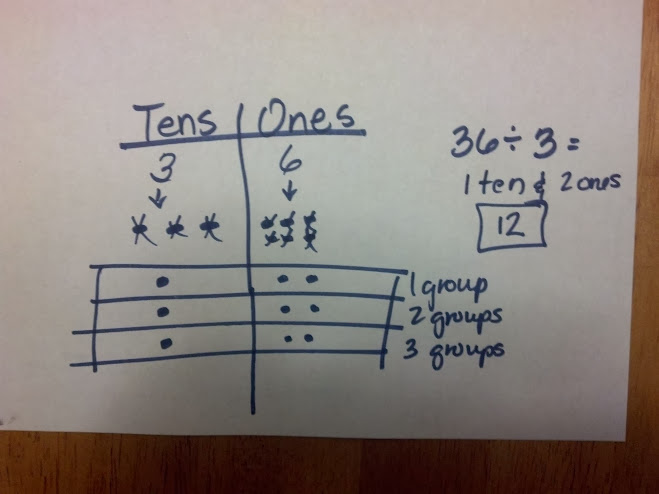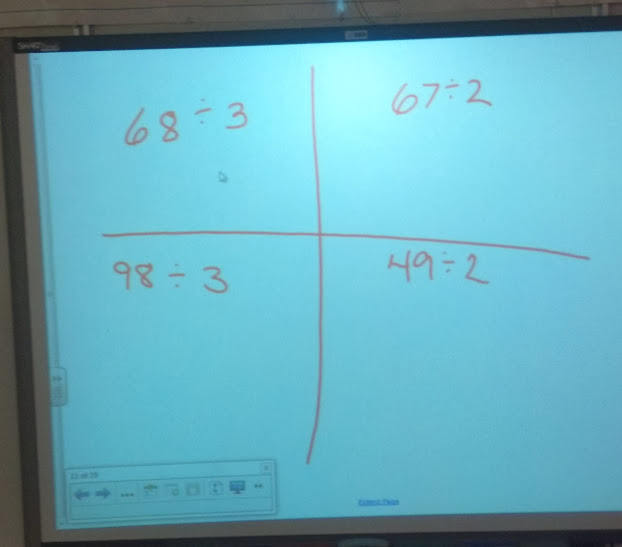# Place Value Chart and Division

12 teachers like this lesson
Print Lesson

## Objective

SWBAT solve two digit dividend division problems with a remainder in the ones place by using a place value chart.

#### Big Idea

Students work towards the unit end goal of dividing muti-digit numbers by a single digit.

## Number Hook

5 minutes

My students really love magic tricks in which they get to use a calculator.
This trick lets each student use a calculator.

A Truly Remarkable Number

Students enter 999999 into their calculator. Then they divide it by seven.
The result will be a mysterious number!

Next, students throw a die (or randomly pick a number from 1 to 6)
and multiply the result by the mysterious number. Then they arrange the
digits of the product from lowest to highest from left to right to form a six-digit number.

What is the number?
All students should get 124578 as their number.

` `

## Warm Up

5 minutes

Being able to multiply and divide fluently within 100 is essential during the early years of schooling, and the basic facts of addition subtraction and multiplication/division are a critical baseline, not only then but also during later work with fractions, decimals, ratio, proportion, and more.

Researchers have gained insights from brain research about demands on the working brain. As students begin to learn math facts, their brains are focused on those basic computations, but as students become automatic with basic facts, their brains are then able to focus on other aspects of the task like the challenges of place value, decimals, or fractions. Being automatic with basic facts frees the brain to focus on other math processes. Committing basic math facts to memory speeds up math tasks. As math tasks increase in complexity, they often require multiple steps to find the solution.

Because basic facts are so important for my students to master, and I have more than half of my class that does not have them mastered, I choose an oral division facts practice for this warm up.  The PowerPoint slides change every 3 seconds. I encourage my students to yell their answers "loud and proud."

For this warm up, students practice basic division facts for numbers divided by three and four.

You can access the power point here: division 3 and 4 practice.ppt

## Concept Development

45 minutes

Students use Math Practice Standard 4 today as they explore how to use a place value chart to model division. I begin this lesson by instructing students to draw a place value chart on their personal white boards. Their place value chart only needs to include ones and tens.

I tell students that for today, we are going to assume we need to find how many groups to make.  I tell students to model on their boards, with me, 6 ÷ 3.  Students should draw 3 groups below.

I ask students questions like; Can we distribute 6 ones into 3 groups? We can think of it like dealing cards or candy evenly among 3 people. First, put one in each group. Cross off the ones one at a time as you distribute them evenly. Next, put another one in each group if you are able. Continue this until all of the ones are distributed.  I ask students if there are any remainders.  I explicitly call the numbers by their names as well, dividend, divisor, quotient and remainder.Next, Display 36 ÷ 3on the board. I ask students what 3 tens and 6 ones represents show. Then I model, along with students, 36 using dots, in the place value chart with 3 dots in the tens to represent 30 and 6 dots in the ones to represent 6. I model how to make room for 3 groups below. I instruct students to begin with the largest unit, in this case the tens. I model how to cross them off to show they are now divided equally into the 3 groups. Next, I model the 6 ones divided by 3. I then ask students how many tens and ones are in each of our 3 groups?Next, I model this same strategy using problems with remainders. I use 5 ÷ 4 and model the four groups with the one remainder.  Then I model  45 ÷ 4 using the same procedure as before.
Then I ask students to show 8 ÷ 3 using the place value chart and dots on their whiteboards while I circulate the room to observe students.  Then students solve 68 ÷ 3.
During this lesson, I am also relating division to multiplication and showing students how multiplication can help them check their work. This allows students to work on Math Practice Standard 2. In order to develop students’ capacity to "reason abstractly and quantitatively" it is imperative that I help  learners understand the relationships between problem scenarios and mathematical representation, as well as how the symbols represent strategies for solution.
For the remainder of the lesson, students solve several more problems like 65 ÷ 2, 47 ÷ 4, etc,  These problems have tens that can be evenly distributed into the groups of tens with a remainder in the ones. In the next lesson, students will decompose tens.
I then assign these problems as homework instructing students to solve using a place value chart. Students fold a piece of paper into fourths and copy the problems onto their paper to bring home as homework.You can see in this video a student explaining the place value chart to divide.